# Getting Started with Machine Learning with Python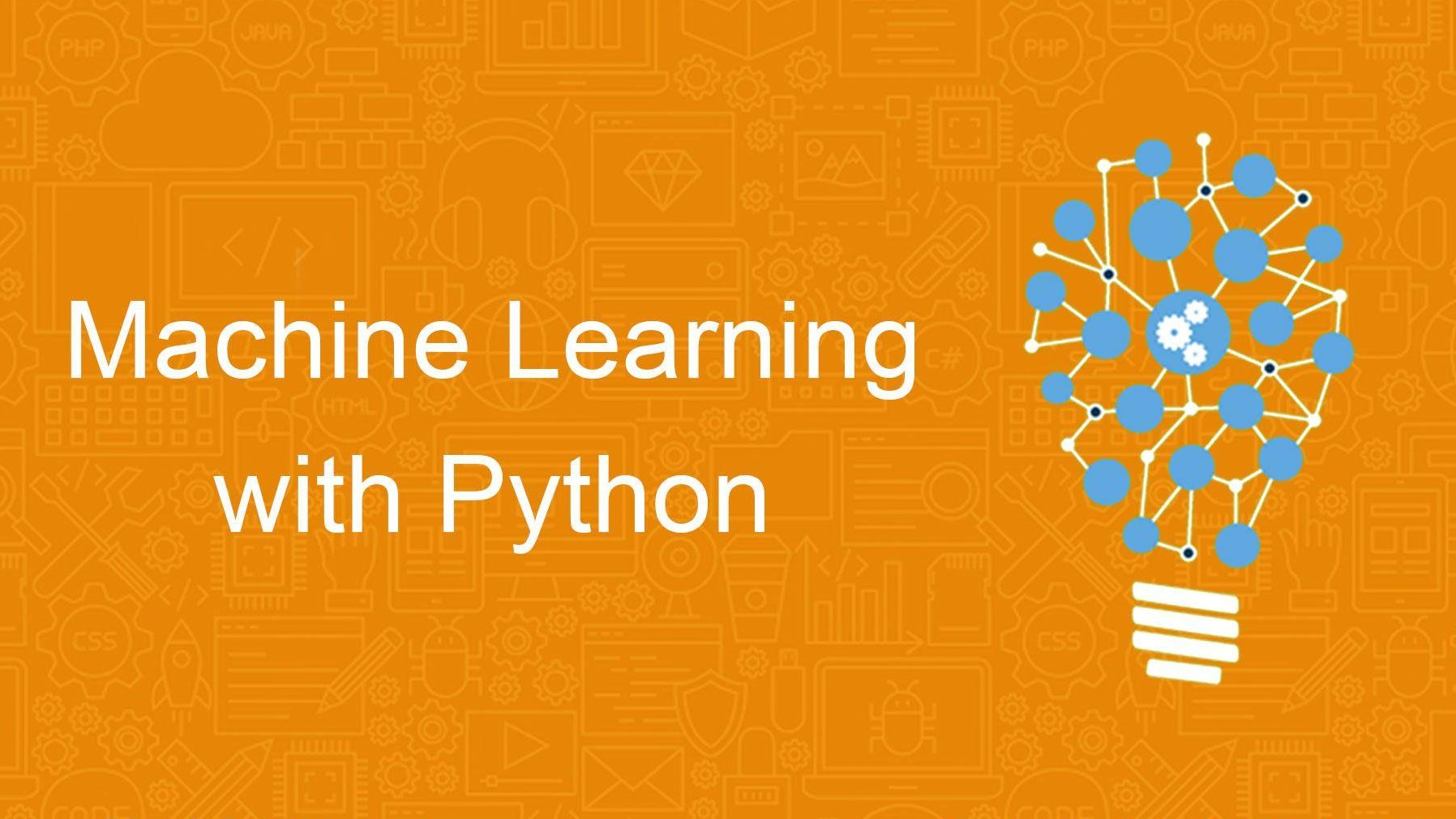### – BY AMAN PANDEY

In my previous tutorial Constructing a Simple Blockchain using PYTHON , I did promise on writing the further about Applications of Blockchain & their Implementation. I will soon post regarding the same. I wanted to make the next tutorial over Blockchain as simple as possible, so I will be needing some time to design my next Tutorial blog on Blockchain. So, keep patience :).

Now, alongside me learning the blockchain, I was also working on Machine Learning and Deep Learning, as if it is my core Learning subjects.

In my last blog on Blockchain, I received few comments that the terms were not easy to understand, thus making the blog difficult to read for the readers completely new to programming, which, is of course very true because these technologies have their own glossary.

I came up with an idea to give you guys the taste of the Machine Learning Models with the easiest way possible, to make my blog better, or you can say that I just trained myself ; P.

# Machine Learning

“Machine learning is a field of computer science that uses statistical techniques to give computer systems the ability to “learn” with data, without being explicitly programmed.”

Does that help?

I guess not!

My belief for doing the things perfectly is by actually doing them.

I would love to dip my hands into something worthy rather than sitting and listening to some boring lectures (though they are not that boring, it’s my way of understanding things 😀 ;p).

So, here I present you the best way, that I think is well enough to get you guys a boost start in making and understanding machine learning models.

## Getting Started

Before actually getting started, let’s get back to the definition and try to understand it,

“Machine learning is a field of computer science that uses statistical techniques to give computer systems the ability to “learn” with data, without being explicitly programmed.”

Few words to underline:

-statistical

-ability to “learn”

-without being explicitly programmed

Now in this tutorial, I will not be taking the names of any technical term except the ones that you need to know. Or better to say the ones which are extremely required. Because I think, for those who are having their first experience in Machine Learning, it becomes extremely confusing when such “Out of their Glossary” kind of terms starts bombarding on them.

So, now how can we start understanding above-underlined terms? How do we actually implement them? How a machine with zero IQ will learn? How will it answer to the problems that are new to them? And most importantly how will we train the machine?

I will try to explain it in very short as I can.

->Statistical means you have previously recorded data of Thousands or millions or even billions of records. E.g. the data of

• Occurrences of words in emails marked as SPAM
• Data of various houses & their degree of damage along with structural information of the houses etc.

These datasets are used to make a Mathematical Model, which will then be used to predict the answers for the test datasets.

->Ability to “learn” here is not that computer gets some human power or something and starts learning on its own. Naah. This the thing which we recently called the Mathematical Model.

We actually create a mathematical model using the previous datasets and train them on basis of them, or in other words to say we actually plot them using various techniques (in fancy words called as Machine Learning Algorithms ) based on features (another fancy term), which actually stands for various properties or information related to some object in which we are going to predict our results on.

E.g.

• Linear Regression
• Logistic Regression
• Decision tree Classifier
• Random Forest
• Neural Networks etc. etc. etc.

😀 Haha.. none of them gives us clue what they mean. Right?

Now before moving forward I would love to illustrate you with some example, you’ll love the way it all works:

Suppose you want to distinguish between an “apple” and an “orange”.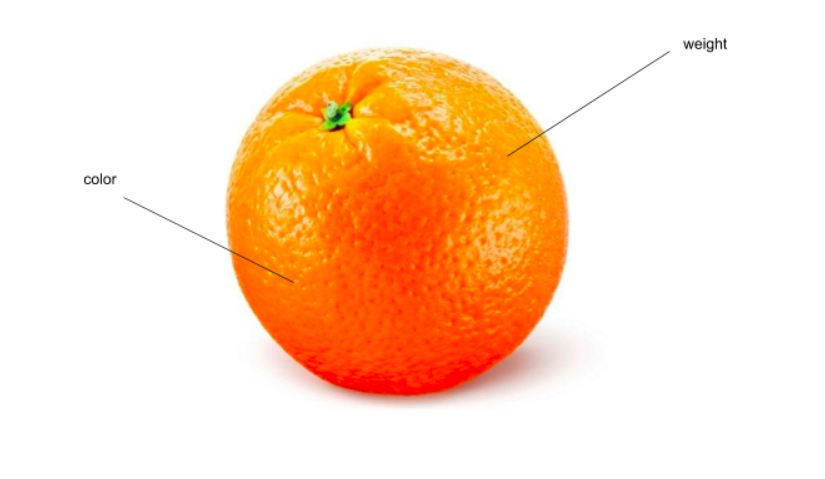Now what you have for information about them?

Ummm, maybe weight, or color may be different levels of its ripeness as it may be possible that apple or orange may have different weights and color at different ripeness level.

Isn’t it?

“Now, we have two features color and weight now.”

A mathematical model is created by plotting these properties on a 2d graph as shown. But that is possible if we have some numerical representation of a feature.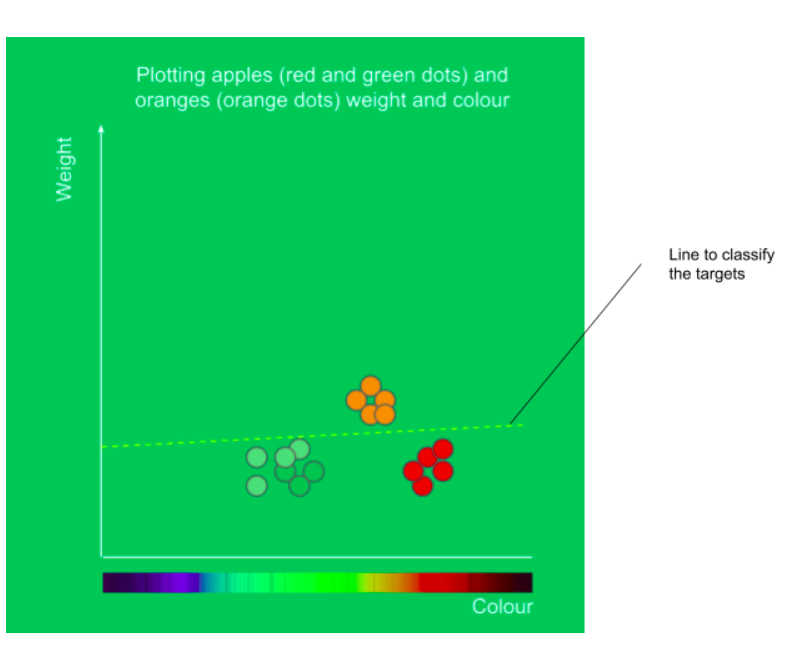In this way, we plot them(intuitively), and ready to classify them.

So for the training data we will plot new inputs on this graph and the examples plotted on this graph having ordinates > line will be oranges and the ones having ordinates <line, are the apples.

This is an example of simple Linear regression, in which we plot a line to classify between two targets.

And this is how a computer performs without being explicitly programmed.

## Why Python?

PYTHON being the most famous language of today, besides another like JAVASCRIPT, is extremely simple, ridiculously fast, and has a huge Library for various uses ranging from COMPUTATIONAL UTILITIES to CREATING A P2P network.

It may happen that you want to do Machine Learning, and you don’t need to take a full course on python. I know many of the sources where you can learn enough python to go on with machine learning.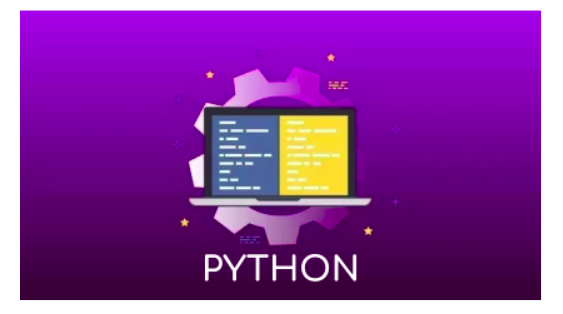## Starting with Building a Machine Learning model.

Steps : –

1. Installing required Libraries
Pandas, scikit learn, numpy… that’s it for now
2. Creating python file, importing required libraries and all
we can do with any library but for now, we’ll just have Iris flower dataset, which is actually considered as “Hello world” dataset for python, you’ll find at many places
4. Exploring our dataset
5. Making our first model
6. Printing the accuracy of our model
7. Testing various models

**Note: Before starting anything I need you to clone the following repo from GitHub link to your local PC:

https://github.com/johnsoncarl/startingWithMachineLearning

## 1. Installing required Libraries

In the Github repo given above, you’ll find a file name required.txt, this file has all the requirements for the project, just run the following command into your terminal, being into repo directory to install required packages.

sudo apt-get -y install python3-pip
pip3 install -r required.txt

This will install all the required libraries for our model.

## 2. Creating a Python file, importing libraries and all

Create a python file of your desired name with .py extension in the repo directory, and open it into your favourite text editor and import required libraries as follows:

```import pandas as pd
from sklearn import model_selection
from sklearn.metrics import accuracy_score
# these are various machine learning models already stored in the sklearn library
from sklearn.linear_model import LogisticRegression
from sklearn.tree import DecisionTreeClassifier
from sklearn.neighbors import KNeighborsClassifier
from sklearn.discriminant_analysis import LinearDiscriminantAnalysis
from sklearn.naive_bayes import GaussianNB
from sklearn.svm import SVC```

Here we shall use read_csv() function of pandas library to read our dataset as follows.

```file = pd.read_csv("iris.data", header = None)

file.head(5) will view the first 5 rows of the dataset.

And do notice, in read_csv we have header = None, this is used because our dataset does not contain any headings to define the columns. It will look something like this: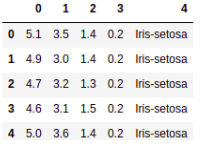## 4. Exploring our dataset

Few things before building our model.

Run the following lines to print various information about the dataset we are going to use.

1. Finding dimensions
`print(file.shape)`

2. Describing data with analytics

`print(file.describe())`

3. Printing distribution of class(grouping according to column no 4, as we have seen in point 3.)

`print(file.groupby(4).size())`

## 5. Making our First model

Before making any Model and testing data on it, we have a very important step, that is to creating training & testing datasets separately. To train the model on and to test the model on.

For this purpose, we have already imported model_selection  from sklearn.

->  Splitting dataset into Training and Testing

Following code is to first change the dataset into a 2D array, then separating the target from it into Y, defining seed. And finally dividing our dataset into training and validation dataset.

```array = file.values     # dataset to a 2d array
X = array[:,0:4]        # feature dataset
Y = array[:,4]          # target dataset
# validation size is used to take out 0.3 i.e 30% of our dataset into test dataset.
validation_size = 0.30
seed = 5                # why random seed is used its given
# finally slicing our dataset into training and testing
X_train, X_validation, Y_train, Y_validation = model_selection.train_test_split(X, Y, test_size=validation_size, random_state=seed)
# to test if its sliced properly
print(X_train[:3])```

-> Defining and using our model

We will be using simple Logistic Regression classifier as our model and use to train our dataset and predict the outcomes.

Few steps, Define model, then fit model, then predict the output.

```model = LogisticRegression()
# fitting our model
model.fit(X_train, Y_train)
# predicting outcomes
predictions = model.predict(X_validation)
print(predictions[:10])```

print(predictions[:10])) will print the predictions on validation dataset after being train on the training dataset.

## 6. Printing the accuracy of our model

Now to rate our model we need to find its accuracy. For this, we need to compare our Validation data to our predicted data. And since we are using a library we don’t need to manually calculate it.  We have the following command to do this job as we have already imported accuracy_score from sklearn.metrics.

`print(accuracy_score(Y_validation, predictions))`

I had the following output when I ran this in my ipython notebook, which I have included in my Github repo.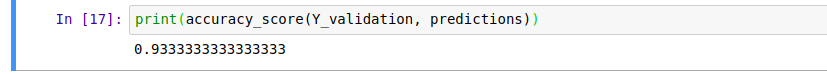It is 93.33% accurate.

And now, you are done with your first machine learning model.

## 7. Testing Various models

```model = LogisticRegression()
model.fit(X_train, Y_train)
predictions = model.predict(X_validation)
print("Logistic Regression: ", accuracy_score(Y_validation, predictions, "\n"))
model = DecisionTreeClassifier()
model.fit(X_train, Y_train)
predictions = model.predict(X_validation)
print("DecisionTreeClassifier: ", accuracy_score(Y_validation, predictions, "\n"))
model = KNeighborsClassifier()
model.fit(X_train, Y_train)
predictions = model.predict(X_validation)
print("KNeigbhorsClassifier: ", accuracy_score(Y_validation, predictions, "\n"))
model = SVC()
model.fit(X_train, Y_train)
predictions = model.predict(X_validation)
print("SVC: ", accuracy_score(Y_validation, predictions, "\n"))
model = LinearDiscriminantAnalysis()
model.fit(X_train, Y_train)
predictions = model.predict(X_validation)
print("LinearDiscriminantAnalysis: ", accuracy_score(Y_validation, predictions, "\n"))
model = GaussianNB()
model.fit(X_train, Y_train)
predictions = model.predict(X_validation)
print("GaussianNB: ", accuracy_score(Y_validation, predictions, "\n"))```

My Output was as Follows: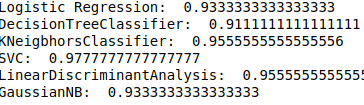Here are various accuracies of different models, we will be learning about in upcoming blogs.

**Please have a look at the ipython nb in the repository. Also, you can comment in the REPOSITORY itself.

So, that’s it with this tutorial blog.

My next blog on Machine Learning will be quite boring as I will be explaining some “Boring” terms of machine learning. And after reading this blog. You’ll have an easy understanding of those terms. And also An intuitive idea of every term if you want to learn good quality machine learning.

***Note. If you want then I’ll be providing some references about it in the blog.

# Please provide your suggestions and even if there’s any doubt regarding whatever you have learned from this blog or any other blog. Just get in touch with me @ my email aman0902pandey@gmail.com.

Or comment it on our FB page or insta page.

Happy Learning!

Cheers….

CEV - Handout